TOP

# Basic Encoding Rules

The Basic Encoding Rules (BER) specify how data should be encoded for transmission, independently of machine type, programming language, or representation within an application program.

### Basic Encoding Rules Overview

BER uses a Tag-Length-Value (TLV) format for encoding information. The type or tag indicates what kind of data follows, the length indicates the length of the data that follows, and the value represents the actual data. Each value may consist of one or more TLV-encoded values, each with its own identifier, length, and contents.

While TLV encodings increase the number of octets transferred, it makes it easier to introduce new fields in messages that can be handled even by older implementations. Another advantage is that the predictability of the encoding makes it easy to debug.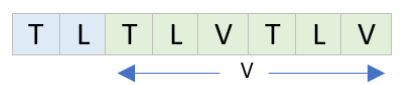### Encoding Identifiers (Tags)

The identifier consists of three parts:

• Class
• 00 universal
• 01 application
• 10 context-specific
• 11 private
• Form
• 0 primitive - is used with types that do not contain other types (INTEGERs and BOOLEANs). The contents octets directly represent the encoded value.
• 1 constructed - is used for types that can include values of other types (SEQUENCEs).
• Number
• 0 <= tag <= 30
In the example below, the identifier occupies only a single octet. Bits 7 and 6, the high order bits, identify the class of the tag being encoded. Bit 5 indicates whether this encoding is primitive or constructed. If bit 5 is 0, the encoding is primitive; if bit 5 is 1, the encoding is constructed. If the number is between 0 and 30 (inclusive), it is encoded in the last five bits of the identifier octet as an unsigned binary integer.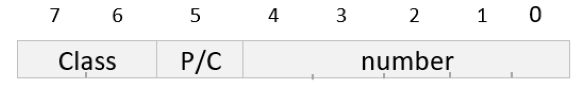• 31 < =tag <= 127
When the number is greater than thirty, the last five bits of this first octet are all set to 1, and the actual value of the tag's number is encoded in one or more following octets. The final octet of this series has bit 7 set to 0.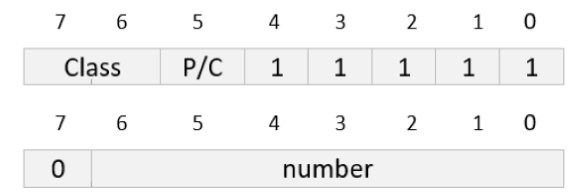• tag > 127
The other octets set bit 7 to 1. The actual value of the tag's number is encoded as an unsigned binary integer, as the concatenation of the rightmost seven bits of each octet.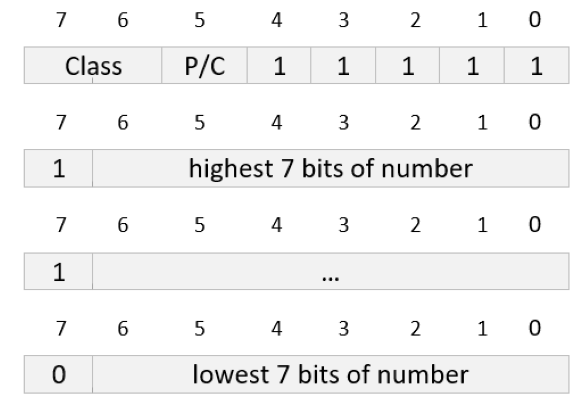#### Examples of Encoded Identifiers

In the following example, BOOLEAN's tag UNIVERSAL 1 is encoded. The encoding is primitive (BOOLEAN contains no other types within it):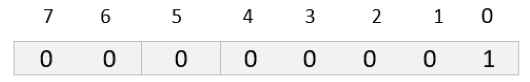In the second example, the tag PRIVATE 13 has a constructed encoding. This tag can be used, for example, to identify a particular ASN.1 SEQUENCE in some proprietary application. Like the first example, the encoded identifier occupies only a single octet: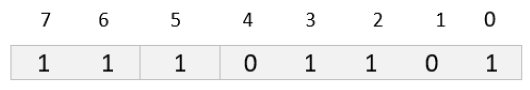The third example shows a more complex case: a primitive encoding of the tag APPLICATION 40. The first octet of the encoding indicates the identifier's class and encoding (primitive or constructed). The last five bits of this first octet, however, are all 1's, indicating that the tag's number is encoded in the following octets. In this case, only one extra octet is required to contain the tag number 40, so bit 7 of this octet is set to 0. The value 40 is encoded in the rightmost seven bits of this extension octet: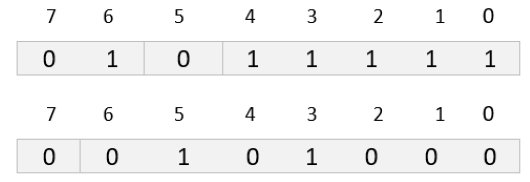### Encoding Lengths

Length is always specified in octets, and includes only the octets in the actual value (the contents). It does not include the lengths of the identifier or of the length field itself.

There are three ways to encode lengths in BER:

• Short form - for lengths between 0 and 127, the one-octet short form can be used. In the encoding below, bit 7 of the length octet is set to 0, and the length is encoded as an unsigned binary value in the octet's rightmost seven bits.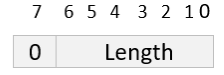• Long form: for lengths between 0 and 21008 octets, the long form can be used. It starts with an octet that contains the length of the length, followed by the actual length of the encoded value. For example, if the first octet of the length contains the value 4, the actual length of the contents is contained in the next four octets. Leading octets that contain all 0's can appear in this second part of a long form length encoding. A sender could, for instance, encode all lengths using the long form, and always represent the actual length in four octets, with a fixed length of the length octet value of 4: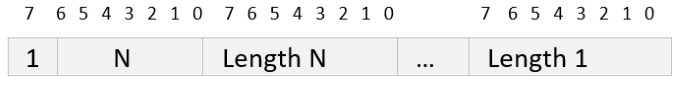• Indefinite form: it is used when the length of the value being encoded is not known at the beginning of the encoding. Or, it may simply be convenient to mark the start and finish of an encoded value rather than include its exact length. In this form, a single ooctet is placed between the identifier and the contents that contain the fixed value 100000002 (hexadecimal 80). The end of the encoded value is indicated by two special end-of-contents octets, each containing all 0's. This special sequence can be thought of as a primitive encoded identifier of UNIVERSAL 0 followed by a length of 0. (No ASN.1 tag is assigned UNIVERSAL 0 specifically to avoid conflict with this form of length encoding.)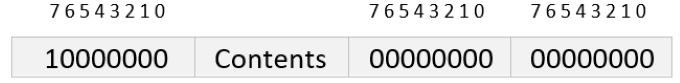#### Examples of Encoded Lengths

In this example, a length of 5 is encoded using the short form. Bit 7 of the single length octet is set to 0, and bits 6-0 contain the binary value 5: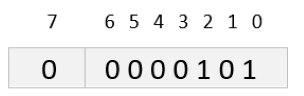The second example shows a long form encoding. Bit 7 of the first octet is set to 1, and bits 6-0 contain the value 2. This indicates that the two following octets contain the actual length of the contents. This length, 1020 octets, is then encoded in binary in the last two octets: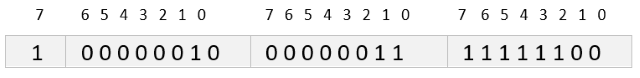In the next example, the long form is used to encode a length of 5. Unlike the previous case, where the short form could not have been used due to the magnitude of the length, the long form is not required here. It is legal, however, to encode any length (up to the maximum) using the long form, and to precede it with any number of leading zero octets. In this example, the first octet of the length has bit 7 set to 1, indicating the long form, and a value of 2 in bits 6-0. This indicates that the following two octets contain the actual length. As the example shows, those two octets consist of one octet of zeros, followed by a 5 encoded in the second octet. A sender can encode all lengths this way to simplify the implementation: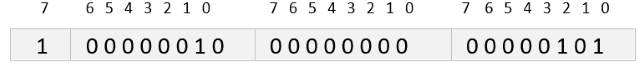The final example shows the indefinite form of encoding, which is used only with constructed encodings; a value using indefinite form is likely to contain other length fields for its embedded values. In the example shown, those embedded lengths all use the short form, but they are free to use any style of length encoding. If one or more of the embedded values has a constructed encoding, it can even use the indefinite form. The length field contains the value 8016, followed by the identifier of the first encoded value within this constructed encoding. The end of the constructed encoding is marked with the end-of-contents indicator, two octets of 0's: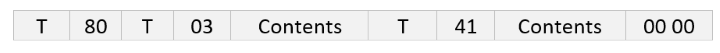#### Example

```Age ::= INTEGER (0..7)
-- 02 01 06```### Encoding Types

##### BOOLEAN
• Values: either TRUE or FALSE.
• A value of all 0's represents FALSE, and any other value represents TRUE.
```operationCompleted BOOLEAN ::= TRUE
-- 01 01 FF```
```Married ::= BOOLEAN
currentStatus Married ::= FALSE
-- 01 01 00```##### INTEGER
• The INTEGER value, positive or negative, is encoded as a 2's complement binary number, with the high order octet in the leftmost (first) position.
• The encoded value can be a single octet or may contain hundreds of octets (although handling large integer values is difficult).
• Leading octets of all 0's (or all 1's) are not allowed. In other words, the leftmost nine bits of an encoded INTEGER value may not be all 0's or all 1's. This ensures that an INTEGER value is encoded in the smallest possible number of octets.
```temperatureToday INTEGER ::= 72
-- 02 01 48```
```Color              ::= INTEGER {red(0), blue(1), yellow(2)}
defaultColor Color ::= blue
-- 02 01 01```##### ENUMERATED
• Each value is encoded as its associated INTEGER value.
```Color ::= ENUMERATED { red (0),
blue  (1),
yellow(2) }
colorOfTheSky Color ::= blue
-- 0A 01 01``````MorP ::= ENUMERATED { minus (-1),
zero  (0),
plus  (1) }
negative MorP ::= minus
-- 0A 01 FF```##### REAL
• Real values are defined according to the formula Value = M * BE. Each of the values M (the mantissa), B (the base), and E (the exponent) must be encoded. M and E may take any positive or negative integer values, while B can take any of the values 2, 8, or 16. Any combination of these values is permitted.
• For encoding purposes the mantissa M is further broken down; M = S * N * 2F, where S is the sign, N the number, and F a binary scaling factor. S can be either 1 or -1, F can be 0, 1, 2, or 3, and N can be any non-negative integer value.

The example shows how the real value 10.0 could be described using this scheme. Note that several different representations of the same real value are possible:

```ten REAL ::= { 10, 2, 0 }
Value = 10 * 20 or 10.0
M = 10
B = 2
E = 0

M = 1 * 10 * 2 0
S = 1
N = 10
F = 0```

Possible contents encoding structure: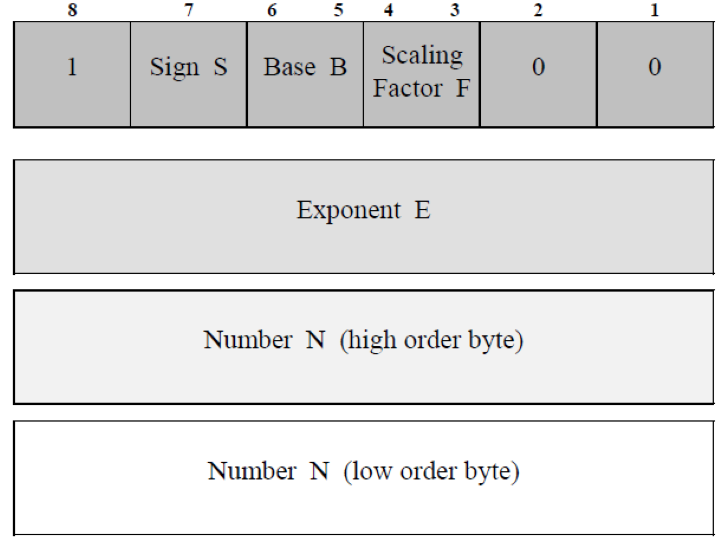```ten REAL ::= { 10, 2, 0 }
-- 09 03 80 00 0A```
##### BIT STRING
• Values may have either primitive or constructed encodings, depending on the encoder. The constructed encoding is used when transmission of the BIT STRING value must begin before the entire value is available (before its length is known).
• Except for a null BIT STRING, the first octet of the contents field indicates the number of unused bits in the last octet of the encoding. Since a BIT STRING value does not need to be an even multiple of eight bits, the first contents octet indicates how many bits are unused in the last octet of the encoded value. Following this is the actual encoded BIT STRING value.

In the first example, the BIT STRING value is 12 bits long. The first octet of the encoded contents contains the value 4, indicating that the last four bits of the final octet are not part of the actual encoded BIT STRING value. The last two octets contain the actual value, padded with zeros to an octet boundary.

```contents2 BIT STRING ::= '101100001001'B
-- 03 03 04 B0 90```

The second example shows a similar structure. Both encodings are primitive:

```Color ::= BIT STRING {red(0),
blue(1),
yellow(2)
}
defaultColors Color ::= {red, yellow}
-- 03 02 05 A0
```The third example shows the special case for a null BIT STRING:

```colorless Color ::= ''B
-- 03 01 00```
##### OCTET STRING
• The encoding can be primitive or constructed (because the length may not be known when transmission begins, a constructed encoding allows the use of indefinite form length).
• The octets of the value are encoded from left to right following the length.

The first OCTET STRING example shows a primitive form encoding of a two-octet value:

```eightBitBytes1 OCTET STRING ::= 'A24F'H
--  04 02 A2 4F```

The second example shows how constructed form encodings are accomplished: the OCTET STRING value is divided into arbitrary length pieces, each encoded as a primitive OCTET STRING. The entire list of these primitive values is then treated as the value (contents) of a single constructed OCTET STRING. This same technique is used when constructed encodings are used with BIT STRING values:

```longString OCTET STRING ::= '00112233445566778899AABBCCDDEEFF'H
--  24 80
--       04 08 00 11 22 33 44 55 66 77
--       04 08 88 99 AA BB CC DD EE FF
--  00 00```
##### NULL
• An identifier, encoding NULL's tag, is followed by a length of 0.
• There are no contents octets.
```currentlyUnknown ::= NULL
-- 05 00```
##### SEQUENCE
• The encodings are always constructed.
• SEQUENCEs are encoded with an identifier, length, and contents. The identifier encodes the SEQUENCE's tag, and the length encodes its length, but the contents are an ordered list of identifier/length/contents encodings, one for each of the SEQUENCE's elements.

In the following example, in an encoding of a value of the SEQUENCE type PersonnelRecord, the SEQUENCE's identifier and length appear first, followed by the SEQUENCE's contents. Those contents, however, are the SEQUENCE's elements, and are encoded as an OCTET STRING value and two INTEGER values. The encoded elements must appear in exactly the order shown in the type definition:

```PersonnelRecord ::= SEQUENCE {
name     OCTET STRING,
location INTEGER { homeOffice(0),
fieldOffice(1),
roving(2)},
age      INTEGER OPTIONAL
}
rockStar1 PersonnelRecord ::= {
name     '6269672068656164'H,
location roving,
age      26}
-- 30 10
--      04 08 62 69 67 20 68 65 61 64
--      02 01 02
--      02 01 1A```##### SEQUENCE OF
• Each element is of the same type.
• A receiver must know the type definition of SEQUENCE OF values to distinguish them from SEQUENCEs and to correctly decode the values.
• The list of elements can be arbitrarily long (unless restricted).
```DailyTemperatures ::= SEQUENCE OF INTEGER
weeklyHighs DailyTemperatures ::= {10, 12, -2, 8}
--  30 0C
--       02 01 0A
--       02 01 0C
--       02 01 FE
--       02 01 08```##### SET
• The encoding is constructed.
• Each element is encoded according to its own rules.
• The elements of a set may appear in any order when encoded.

In the following example, the elements location and age are encoded in reverse order from that specified in the type definition. This flexibility in encoding is what requires the context-specific tags to be added to the elements of the SET:

```PersonnelRecord ::=  SET {
name  OCTET STRING,
location  INTEGER {
homeOffice(0),
fieldOffice(1),
roving(2)
},
age   INTEGER OPTIONAL
}
rockStar3 PersonnelRecord ::= {
name    '44617679204A6F6E6573'H,
location homeOffice,
age 44
}
--  A0 12
--       80 0A 44 61 76 79 20 4A 6F 6E 65 73
--       82 01 2C
--       81 01 00```##### CHOICE
• A value derived from a CHOICE type is encoded according to the rules for the chosen type.

In the following example, any value of type Division will be either the SEQUENCE type named manufacturing or the SEQUENCE type named r-and-d. In the value specified, r-and-d is chosen, therefore, the encoding is that of the SEQUENCE defined for r-and-d:

```Division ::= CHOICE {
manufacturing      SEQUENCE {
plantID      INTEGER,
majorProduct OCTET STRING}
r-and-d                       SEQUENCE {
labID          INTEGER,
currentProject OCTET STRING
}
currentAssignment Division ::=
r-and-d : { labID 48,
currentProject '44582D37'H}
--  A1 09
--       02 01 30
--       04 04 44 58 2D 37```##### Character String Values
• The encodings of each character string type, including NumericString, PrintableString, and GraphicString, are derived from OCTET STRING.
• Any character string value can have either a primitive or constructed encoding.
• For character set types that allow values from several different character sets, the character set in use must be identified by an appropriate escape sequence in the encoded value. With some types, the same character value can have more than one legal encoding, depending on which character set is chosen.
```targetSales NumericString ::= "1000000"
--  12 07 31 30 30 30 30 30 30

topAuthor PrintableString ::= "Parker"
--  13 06 50 61 72 6B 65 72

letters UTF8String ::= "abcdlmyz"
--  0C 08 61 62 63 64 6C 6D 79 7A```
##### OBJECT IDENTIFIER and ObjectDescriptor
• The numbers in the OBJECT IDENTIFIER value (each is called a "subidentifier" in the BER standard) are represented as binary integers, but the encoding form for INTEGER is not used. Instead, each subidentifier is represented as a series of octets. In all except for the last octet, bit 8 is set to 1; the 0 value of bit 8 in the last octet indicates the end of this subidentifier (number). The integer value of the subidentifier is encoded in the remaining bits of each octet. The resulting value is the concatenation of the rightmost seven bits in the octets comprising a subidentifier. (Note that this is the same convention used for encoding large tag numbers into identifiers.)
• Because the object identifier tree has only a limited number of branches near the top, an optimization is used when encoding the first two subidentifiers. Instead of following the scheme described above, the first two numbers in an OBJECT IDENTIFIER value are encoded in a single octet. The number to be encoded is derived using the formula (X*40) + Y, where X is the value of the first subidentifier and Y the value of the second. In the example, X is equal to 1 (the branch labeled iso) and Y is equal to 0 (the branch under iso(1) labeled standard). The value encoded in the first octet, then, is (1*40) + 0, or 40 (decimal).
• ObjectDescriptors are encoded as retagged GraphicStrings.
• GraphicString's tag is replaced with the UNIVERSAL 7 tag assigned to ObjectDescriptor, and the characters making up the ObjectDescriptor value are represented according to the encoding specified for that character set.
```ftam1 OBJECT IDENTIFIER ::= { iso standard 8571 abstract-syntax(2) ftam-pci(1) }
--  06 05 28 C2 7B 02 01

ftamPDUs ObjectDescriptor ::= "FTAM PCI"
-- 07 08 46 54 41 4D 20 50 43 49```
##### Time Types
```endOfTime DATE ::= "2012-12-21"
-- 1F 1F 08 32 30 31 32 31 32 32 31

zero DATE-TIME ::= "1951-10-14T15:30:00"
--  1F 21 31 39 35 31 31 30 31 34
--  31 35 33 30 30 30

millenium DURATION ::= "P1000Y"
--  1F 22 05 31 30 30 30 59

dawn TIME-OF-DAY ::= "06:30:00"
--  1F 20 06 30 36 33 30 30 30```